Anúncio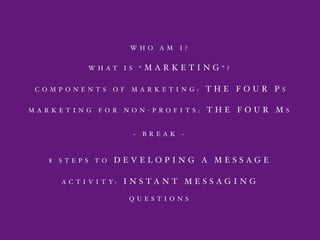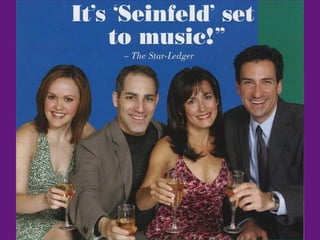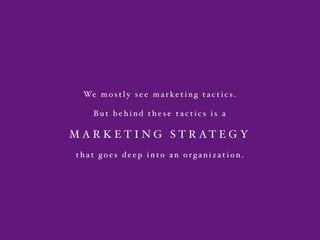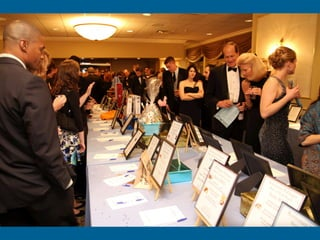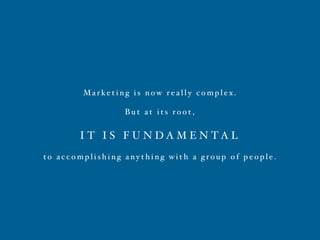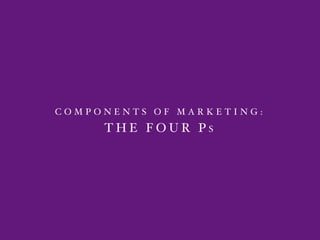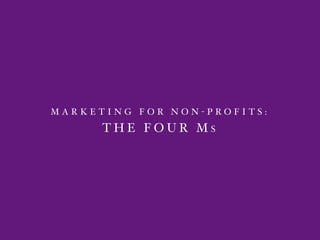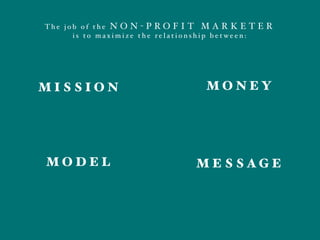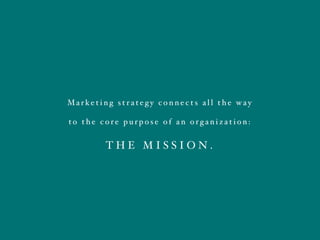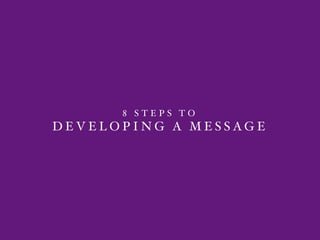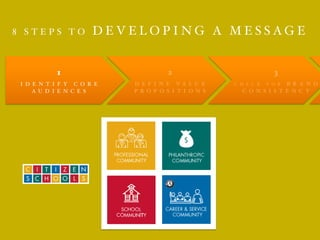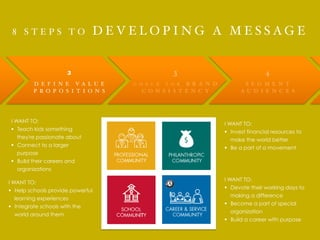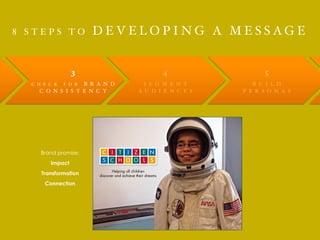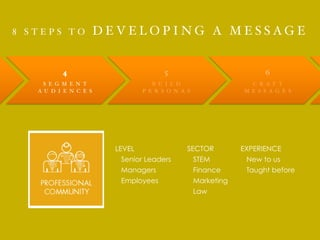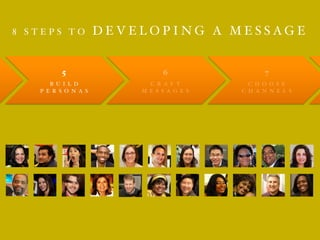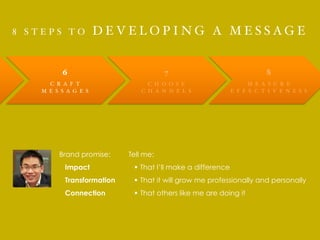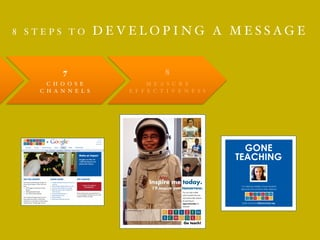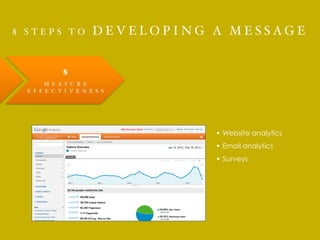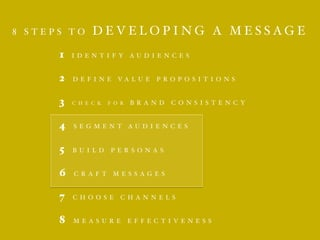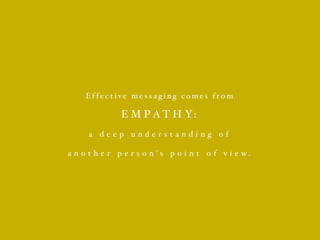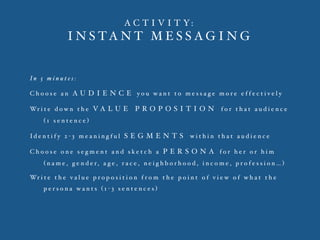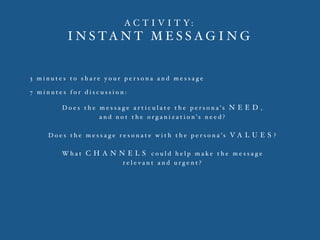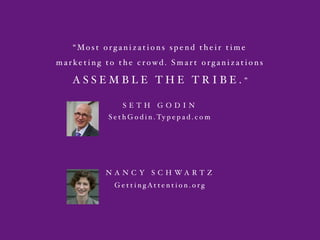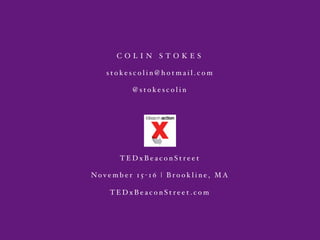1 de 42
Anúncio

### Non-profit marketing workshop

1. W H O A M I ? W H A T I S “ M A R K E T I N G ” ? C O M P O N E N T S O F M A R K E T I N G : T H E F O U R P S M A R K E T I N G F O R N O N - P R O F I T S : T H E F O U R M S ~ B R E A K ~ 8 S T E P S T O D E V E L O P I N G A M E S S A G E A C T I V I T Y : I N S T A N T M E S S A G I N G Q U E S T I O N S
2. W H O A M I ?
3. W H A T I S “ M A R K E T I N G ” ?
4. We m o s t l y s e e m a r ke t i n g t a c t i c s . B u t b e h i n d t h e s e t a c t i c s i s a M A R K E T I N G S T R A T E G Y t h a t g o e s d e e p i n t o a n o r g a n i z a t i o n .
5. Ma r ke t i n g i s n o w r e a l l y c o m p l e x . B u t a t i t s r o o t , I T I S F U N D A M E N T A L t o a c c o m p l i s h i n g a n y t h i n g w i t h a g r o u p o f p e o p l e .
6. C O M P O N E N T S O F M A R K E T I N G : T H E F O U R P S
7. PRODUCT
8. PLACE
9. PRICE
10. PROMOTION
11. M A R K E T I N G F O R N O N - P R O F I T S : T H E F O U R M S
12. PRODUCTPRODUCTMISSION
13. PLACEPLACEMODEL
14. PRICEPRICEMONEY
15. PROMOTIONPROMOTIONMESSAGE
16. T h e j o b o f t h e N O N - P R O F I T M A R K E T E R i s t o m a x i m i z e t h e r e l a t i o n s h i p b e t w e e n : M O N E Y M E S S A G E M I S S I O N M O D E L
17. Ma r ke t i n g s t r a t e g y c o n n e c t s a l l t h e w a y t o t h e c o r e p u r p o s e o f a n o r g a n i z a t i o n : T H E M I S S I O N .
18. ~ B R E A K ~
19. 8 S T E P S T O D E V E L O P I N G A M E S S A G E
20. 8 S T E P S T O D E V E L O P I N G A M E S S A G E 1 I D E N T I F Y C O R E A U D I E N C E S 2 D E F I N E V A L U E P R O P O S I T I O N S 3 C H E C K F O R B R A N D C O N S I S T E N C Y
21. 8 S T E P S T O D E V E L O P I N G A M E S S A G E 2 D E F I N E V A L U E P R O P O S I T I O N S 3 C H E C K F O R B R A N D C O N S I S T E N C Y 4 S E G M E N T A U D I E N C E S I WANT TO: §  Teach kids something they're passionate about §  Connect to a larger purpose §  Build their careers and organizations I WANT TO: §  Invest financial resources to make the world better §  Be a part of a movement I WANT TO: §  Help schools provide powerful learning experiences §  Integrate schools with the world around them I WANT TO: §  Devote their working days to making a difference §  Become a part of special organization §  Build a career with purpose
22. 8 S T E P S T O D E V E L O P I N G A M E S S A G E 3 C H E C K F O R B R A N D C O N S I S T E N C Y 4 S E G M E N T A U D I E N C E S 5 B U I L D P E R S O N A S Helping all children discover and achieve their dreams Brand promise: Impact Transformation Connection
23. 8 S T E P S T O D E V E L O P I N G A M E S S A G E 4 S E G M E N T A U D I E N C E S 5 B U I L D P E R S O N A S 6 C R A F T M E S S A G E S LEVEL Senior Leaders Managers Employees SECTOR STEM Finance Marketing Law EXPERIENCE New to us Taught before
24. 8 S T E P S T O D E V E L O P I N G A M E S S A G E 5 B U I L D P E R S O N A S 6 C R A F T M E S S A G E S 7 C H O O S E C H A N N E L S COMMUNITY COMMUNITY
25. 8 S T E P S T O D E V E L O P I N G A M E S S A G E 6 C R A F T M E S S A G E S 7 C H O O S E C H A N N E L S 8 M E A S U R E E F F E C T I V E N E S S Tell me: §  That I’ll make a difference §  That it will grow me professionally and personally §  That others like me are doing it Brand promise: Impact Transformation Connection
26. 8 S T E P S T O D E V E L O P I N G A M E S S A G E 7 C H O O S E C H A N N E L S 8 M E A S U R E E F F E C T I V E N E S S GONE TEACHING I’m helping middle school students discover and achieve their dreams. Learn more at citizenschools.org.
27. 8 S T E P S T O D E V E L O P I N G A M E S S A G E 8 M E A S U R E E F F E C T I V E N E S S §  Website analytics §  Email analytics §  Surveys
28. 8 S T E P S T O D E V E L O P I N G A M E S S A G E 1 I D E N T I F Y A U D I E N C E S 2 D E F I N E V A L U E P R O P O S I T I O N S 3 C H E C K F O R B R A N D C O N S I S T E N C Y 4 S E G M E N T A U D I E N C E S 5 B U I L D P E R S O N A S 6 C R A F T M E S S A G E S 7 C H O O S E C H A N N E L S 8 M E A S U R E E F F E C T I V E N E S S
29. Ef f e c t i v e m e s s a g i n g c o m e s f r o m E M P A T H Y: a d e e p u n d e r s t a n d i n g o f a n o t h e r p e r s o n ’ s p o i n t o f v i e w .
30. A C T I V I T Y: I N S T A N T M E S S A G I N G
31. A C T I V I T Y: I N S T A N T M E S S A G I N G I n 5 m i n u t e s : C h o o s e a n A U D I E N C E y o u w a n t t o m e s s a g e m o r e e f f e c t i v e l y Wr i t e d o w n t h e V A L U E P R O P O S I T I O N f o r t h a t a u d i e n c e ( 1 s e n t e n c e ) Id e n t i f y 2 - 3 m e a n i n g f u l S E G M E N T S w i t h i n t h a t a u d i e n c e C h o o s e o n e s e g m e n t a n d s k e t c h a P E R S O N A f o r h e r o r h i m ( n a m e , g e n d e r, a g e , r a c e , n e i g h b o r h o o d , i n c o m e , p r o f e s s i o n … ) Wr i t e t h e v a l u e p r o p o s i t i o n f r o m t h e p o i n t o f v i e w o f w h a t t h e p e r s o n a w a n t s ( 1 - 3 s e n t e n c e s )
32. A C T I V I T Y: I N S T A N T M E S S A G I N G 3 m i n u t e s t o s h a r e y o u r p e r s o n a a n d m e s s a g e 7 m i n u t e s f o r d i s c u s s i o n : D o e s t h e m e s s a g e a r t i c u l a t e t h e p e r s o n a ’ s N E E D , a n d n o t t h e o r g a n i z a t i o n ’ s n e e d ? D o e s t h e m e s s a g e r e s o n a t e w i t h t h e p e r s o n a ’ s V A L U E S ? W h a t C H A N N E L S c o u l d h e l p m a k e t h e m e s s a g e r e l e v a n t a n d u r g e n t ?
33. Q U E S T I O N S
34. “Mo s t o r g a n i z a t i o n s s p e n d t h e i r t i m e m a r ke t i n g t o t h e c r o w d . S m a r t o r g a n i z a t i o n s A S S E M B L E T H E T R I B E . ” S E T H G O D I N S e t h G o d i n . Ty p e p a d . c o m N A N C Y S C H W A R T Z G e t t i n g At t e n t i o n . o r g
35. C O L I N S T O K E S s t o k e s c o l i n @ h o t m a i l . c o m @ s t o k e s c o l i n T E D x B e a c o n S t r e e t No v e m b e r 1 5 - 1 6 | B r o o k l i n e , M A T E D x B e a c o n S t r e e t . c o m
Anúncio